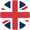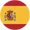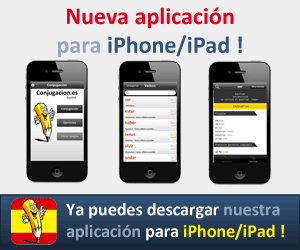# Conjugación del verbo inglés to quizto quiz ➔cuestionar

## Indicative

I quiz
you quiz
he quizes
we quiz
you quiz
they quiz

I am quizing
you are quizing
he is quizing
we are quizing
you are quizing
they are quizing

I quized
you quized
he quized
we quized
you quized
they quized

### Past progressive/continuous

I was quizing
you were quizing
he was quizing
we were quizing
you were quizing
they were quizing

I have quized
you have quized
he has quized
we have quized
you have quized
they have quized

### Present perfect progressive/continuous

I have been quizing
you have been quizing
he has been quizing
we have been quizing
you have been quizing
they have been quizing

### Future

I will/shall quiz
you will quiz
he will quiz
we will/shall quiz
you will quiz
they will quiz

### Future progressive/continuous

I will/shall be quizing
you will be quizing
he will be quizing
we will/shall be quizing
you will be quizing
they will be quizing

### Future perfect

I will/shall have quized
you will have quized
he will have quized
we will/shall have quized
you will have quized
they will have quized

### Future perfect continuous

I will/shall have been quizing
you will have been quizing
he will have been quizing
we will/shall have been quizing
you will have been quizing
they will have been quizing

## Conditional

### Simple

I would/should quiz
you would quiz
he would quiz
we would/should quiz
you would quiz
they would quiz

### Progressive

I would/should be quizing
you would be quizing
he would be quizing
we would/should be quizing
you would be quizing
they would be quizing

### Perfect

I would/should have quized
you would have quized
he would have quized
we would/should have quized
you would have quized
they would have quized

### Perfect progressive

I would/should have been quizing
you would have been quizing
he would have been quizing
we would/should have been quizing
you would have been quizing
they would have been quizing

Otros verbos